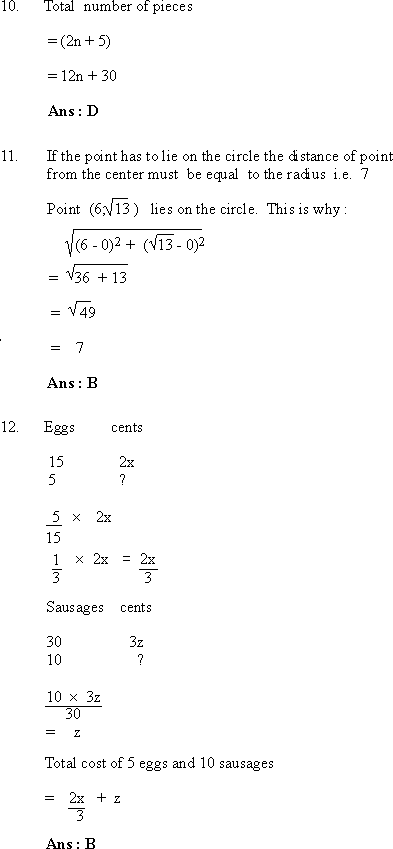Your browser does not support the IFRAME tag.Home MonkeyNotes Printable Notes Digital Library Study Guides Study Smart Parents Tips College Planning Test Prep Fun Zone Help / FAQ How to Cite New Title RequestLength of the uncommon region if least overlap occurs = Circumference - Length of the greater arc = 30 - 24 = 6 Length of the least overlap region = 16 - 6 = 10 Ans : B Painted area = Area of four walls 100 = 4x2 x2 = 25 where x is side of square x = 5 Volume of cube = x3 = 5 ´ 5 ´ 5 = 125 Ans : D Let the integers be 3x and 2x. 3x - 2x = 36 x = 36 The smallest integer is 72. Ans : A Since the line segment from B to A is broken, the Pythagorean method for determining length BA cannot be used. Also since each segment is parallel either to OA or OB, the total length from B to A must necessarily be (a + g). Ans : E Index PART IV Drill : Section 1 : Verbal Reasoning Section 2 : Mathematical Reasoning Section 3 : Verbal Reasoning Section 4 : Mathematical Reasoning Section 5 : Reading Comprehension Section 6 : Mathematical Reasoning Section 7 : Verbal Reasoning Answer Explanation To The Drill Section 1 : Verbal Reasoning Section 2 : Mathematical Reasoning Section 3 : Verbal Reasoning Section 4 : Mathematical Reasoning Section 5 : Reading Comprehension Section 6 : Mathematical Reasoning Section 7 : Verbal Reasoning Test 1
 Search: All Products Books Popular Music Classical Music Video DVD Toys & Games Electronics Software Tools & Hardware Outdoor Living Kitchen & Housewares Camera & Photo Cell Phones Keywords: